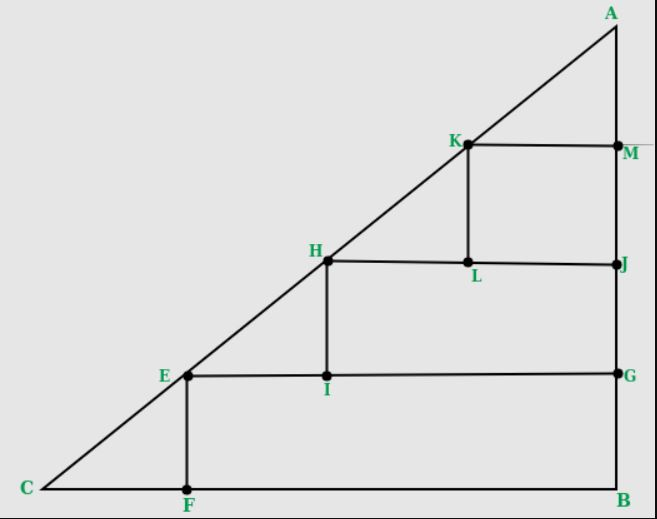# Maximum number of 2×2 squares that can be fit inside a right isosceles triangle

• Difficulty Level : Medium
• Last Updated : 20 Jun, 2022

What is the maximum number of squares of size 2×2 units that can be fit in a right-angled isosceles triangle of a given base (in units).
A side of the square must be parallel to the base of the triangle.

Examples:

```Input  : 8
Output : 6
Please refer below diagram for explanation.``````Input : 7
Output : 3```

Since the triangle is isosceles, the given base would also be equal to the height. Now in the diagonal part, we would always need an extra length of 2 units in both height and base of the triangle to accommodate a triangle. (The CF and AM segment of the triangle in the image. The part that does not contribute to any square). In the remaining length of base, we can construct length / 2 squares. Since each square is of 2 units, same would be the case of height, there is no need to calculate that again.
So, for each level of given length we can construct “(length-2)/2” squares. This gives us a base of “(length-2)” above it. Continuing this process to get the no of squares for all available “length-2” height, we can calculate the squares.

```while length > 2
answer += (length - 2 )/2
length = length - 2```

For more effective way, we can use the formula of sum of AP n * ( n + 1 ) / 2, where n = length – 2

## C++

 `// C++ program to count number of 2 x 2``// squares in a right isosceles triangle``#include``using` `namespace` `std;` `int` `numberOfSquares(``int` `base)``{``   ``// removing the extra part we would``   ``// always need``   ``base = (base - 2);` `   ``// Since each square has base of``   ``// length of 2``   ``base = ``floor``(base / 2);` `   ``return` `base * (base + 1)/2;``}` `// Driver code``int` `main()``{``   ``int` `base = 8;``   ``cout << numberOfSquares(base);``   ``return` `0;``}``// This code is improved by heroichitesh.`

## Java

 `// Java program to count number of 2 x 2``// squares in a right isosceles triangle` `class` `Squares``{``  ``public` `static`  `int` `numberOfSquares(``int` `base)``   ``{``      ``// removing the extra part``      ``// we would always need``      ``base = (base - ``2``);`` ` `      ``// Since each square has``      ``// base of length of 2``      ``base = Math.floorDiv(base, ``2``);`` ` `      ``return` `base * (base + ``1``)/``2``;``   ``}`` ` `   ``// Driver code``   ``public` `static` `void` `main(String args[])``   ``{``      ` `      ``int` `base = ``8``;``      ``System.out.println(numberOfSquares(base));``   ``}``}` `// This code is contributed by Anshika Goyal and improved by heroichitesh.`

## Python3

 `# Python3 program to count number``# of 2 x 2 squares in a right``# isosceles triangle``def` `numberOfSquares(base):``    ` `    ``# removing the extra part we would``    ``# always need``    ``base ``=` `(base ``-` `2``)``    ` `    ``# Since each square has base of``    ``# length of 2``    ``base ``=` `base ``/``/` `2``    ` `    ``return` `base ``*` `(base ``+` `1``) ``/` `2``    ` `# Driver code``base ``=` `8` `print``(numberOfSquares(base))` `# This code is contributed by Anant Agarwal and improved by heroichitesh.`

## C#

 `// C# program to count number of 2 x 2``// squares in a right isosceles triangle``using` `System;` `class` `GFG {``    ` `    ``public` `static` `int` `numberOfSquares(``int` `_base)``    ``{``        ` `        ``// removing the extra part``        ``// we would always need``        ``_base = (_base - 2);``    ` `        ``// Since each square has``        ``// base of length of 2``        ``_base = _base / 2;``    ` `        ``return` `_base * (_base + 1)/2;``    ``}``    ` `    ``// Driver code``    ``public` `static` `void` `Main()``    ``{``        ` `        ``int` `_base = 8;``        ``Console.WriteLine(numberOfSquares(_base));``    ``}``}` `// This code is contributed by anuj_67.`

## PHP

 ``

## Javascript

 ``

Output:

`6`

Time complexity : O(1)

Auxiliary Space : O(1)

This article is contributed by Harshit Agrawal. If you like GeeksforGeeks and would like to contribute, you can also write an article using write.geeksforgeeks.org or mail your article to review-team@geeksforgeeks.org. See your article appearing on the GeeksforGeeks main page and help other Geeks.